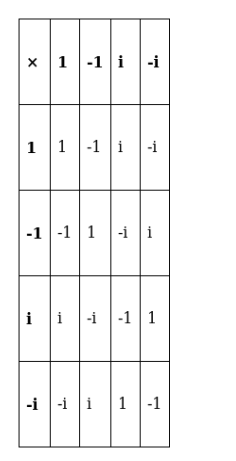# Let A = (1, -1, i, -i) be the set of four 4th roots of unity.Question:

Let A = (1, -1, i, -i) be the set of four 4th roots of unity. Prepare the composition table for multiplication on A and show that

(i) $A$ is closed for multiplication,

(ii) multiplication is associative on $A$,

(iii) multiplication is commutative on $A$,

(iv) 1 is the multiplicative identity,

(v) every element in A has its multiplicative inverse.

Solution:

(i) A is said to be closed on * if all the elements of a*b ∈A. composition table is$\left(\operatorname{as} i^{2}=-1\right)$

As table contains all elements from set A, A is close for multiplication operation.

(ii) For associative, $a \times(b \times c)=(a \times b) \times c$

$1 \times(-i \times i)=1 \times 1=1$

$(1 \times-i) \times i=-i \times i=1$

$a \times(b \times c)=(a \times b) \times c$, so $A$ is associative for multiplication.

(iii) For commutative, $a \times b=b \times a$

$1 \times-1=-1$

$-1 \times 1=-1$

$a \times b=b \times a$, so $A$ is commutative for multiplication.

(iv) For multiplicative identity element $e, a \times e=e \times a=a$ where $a \in A$.

a× e = a

a(e-1) = 0

either a = 0 or e = 1 as a≠0 hence e = 1

So, multiplicative identity element e = 1.

(v) For multiplicative inverse of every element of $A, a * b=e$ where $a, b \in A$.

$1 \times \mathrm{b}_{1}=1$

$b_{1}=1$

$-1 \times b_{2}=1$

$b_{2}=-1$

$\mathrm{i} \times \mathrm{b}_{3}=1$

$\mathrm{b}_{3}=\frac{1}{\mathrm{i}} \Rightarrow \frac{1}{\mathrm{i}} \times \frac{\mathrm{i}}{\mathrm{i}}=\frac{\mathrm{i}}{\mathrm{i}^{2}} \Rightarrow \frac{\mathrm{i}}{-1}=-\mathrm{i}$

$-\mathrm{i} \times \mathrm{b}_{4}=1$

$\mathrm{b}_{4}=\frac{1}{-\mathrm{i}} \Rightarrow \frac{1}{-\mathrm{i}} \times \frac{\mathrm{i}}{\mathrm{i}}=\frac{\mathrm{i}}{-\mathrm{i}^{2}} \Rightarrow \frac{\mathrm{i}}{-(-1)}=\mathrm{i}$

So, multiplicative inverse of $A=\{1,-1,-i, i\}$